## Practical Machine Learning To Estimate Traffic Flow In San Juan, Puerto Rico.

### Round 1: Object Detection? YOLOv5!

Getting started with out a pile of data can make building models difficult. We needed a nice way to collect some data from the traffic here in San Juan and found DTOP has some strategically located webcams strung along the main highway cutting through the city. Great! Except this isn't particularly useful and while manually cleaning data is always on the table, it's not something I want to do for each new image coming through the feed. yolov5 to the rescue!

YoloV5 is an object detection model `ultralytics/yolov5` that is impressively accurate out of the box with identifying things we care about. What do we care about? Cars, buses and trucks in particular. So let's build a model and start feeding it input from our webcams around the city. We're using PyTorch here, this approach is fairly agnostic, but we like pytorch.

```                    ```
import json
from PIL import Image
import numpy as np
import torch
import time
device = torch.device("cuda:0" if torch.cuda.is_available() else "cpu")

model.to(device)

# Our 7 cameras to care about at the moment
sj1 = ("http://its.dtop.gov.pr/images/cameras/26-0.1_01_MD-IPV.jpg",
"http://its.dtop.gov.pr/en/TrafficImage.aspx?id=119&Large=1", 10, 25, 18.458339088897567, -66.08570387019088)
sj2 = ("http://its.dtop.gov.pr/images/cameras/26-1.1_03_MD-IPV.jpg",
"http://its.dtop.gov.pr/en/TrafficImage.aspx?id=121&Large=1", 10, 25, 18.454611048837556, -66.07684808241595)
sj3 = ("http://its.dtop.gov.pr/images/cameras/26-2.1_04_WB-IPV.jpg",
"http://its.dtop.gov.pr/en/TrafficImage.aspx?id=143&Large=1", 10, 25, 18.451301357824246, -66.0680360794367)
sj4 = ("http://its.dtop.gov.pr/images/cameras/26-3.0_05_MD-IPV.jpg",
"http://its.dtop.gov.pr/en/TrafficImage.aspx?id=123&Large=1", 20, 35, 18.44865740439453, -66.06059797491021)
sj5 = ("http://its.dtop.gov.pr/images/cameras/26-5.7_07_WB-IPV.jpg",
"http://its.dtop.gov.pr/en/TrafficImage.aspx?id=125&Large=1", 10, 25, 18.44634309886566, -66.03470036662318)
sj6 = ("http://its.dtop.gov.pr/images/cameras/26-6.5_08_WB-IPV.jpg",
"http://its.dtop.gov.pr/en/TrafficImage.aspx?id=126&Large=1", 20, 30, 18.443494845651536, -66.02780514096465)
sj7 = ("http://its.dtop.gov.pr/images/cameras/CCTV_Minillas_PR-22.jpg",
"http://its.dtop.gov.pr/en/TrafficImage.aspx?id=57&Large=1", 16, 25, 18.44818055202958, -66.06798119910
sjOriRoutes = [sj1, sj2, sj3, sj4, sj5, sj6,
class NpEncoder(json.JSONEncoder):
def default(self, obj):
if isinstance(obj, np.integer):
return int(obj)
if isinstance(obj, np.floating):
return float(obj)
if isinstance(obj, np.ndarray):
return obj.tolist()
return super(NpEncoder, self).default(
def printTraffic(carCount, route):
if carCount == 0:
return "no traffic"
if carCount < route:
return "low traffic"
if carCount < route:
return "medium traffic"
return "high traf
starttime = time.ti
# every 60 seconds let's go grab the next batch of cam images
# ask our model for detections
# count them
# save our file and results for an app to grab
while True:
finalResult = []
for route in sjOriRoutes:
results = model(route)
counts = results.pandas().xyxy.name.value_counts()
vehicleCount = 0
if "car" in counts:
vehicleCount += counts["car"]
if "bus" in counts:
vehicleCount += counts["bus"]
if "truck" in counts:
vehicleCount += counts["truck"]
res = printTraffic(vehicleCount, route)
results.render()
fileName = "static/img/"+route.split('/')[-1]
finalResult.append(
(fileName, route, route, route, route, vehicleCount, res, route, route))
im = Image.fromarray(results.imgs)
im.save(fileName)
with open('latest.json', 'w') as outfile:
json.dump(finalResult, outfile, cls=NpEncoder)
time.sleep(60)
```
```

So we have a fairly intuitive way of grabbing vehicles, counting them out and with some arbitrary numbers, decide if it's no/low/medium or high traffic in the image. However it didn't take long to realize webcam quaility isn't great and the object detection fails to detect all the cars all the time, leaving us with a full freeway being marked as medium or even low traffic. This wasn't the worst offenses though, at night time we realized a packed rush hour ride home was failing to find a single car in the sea of brake lights, so grid lock traffic was decidedly "No traffic"....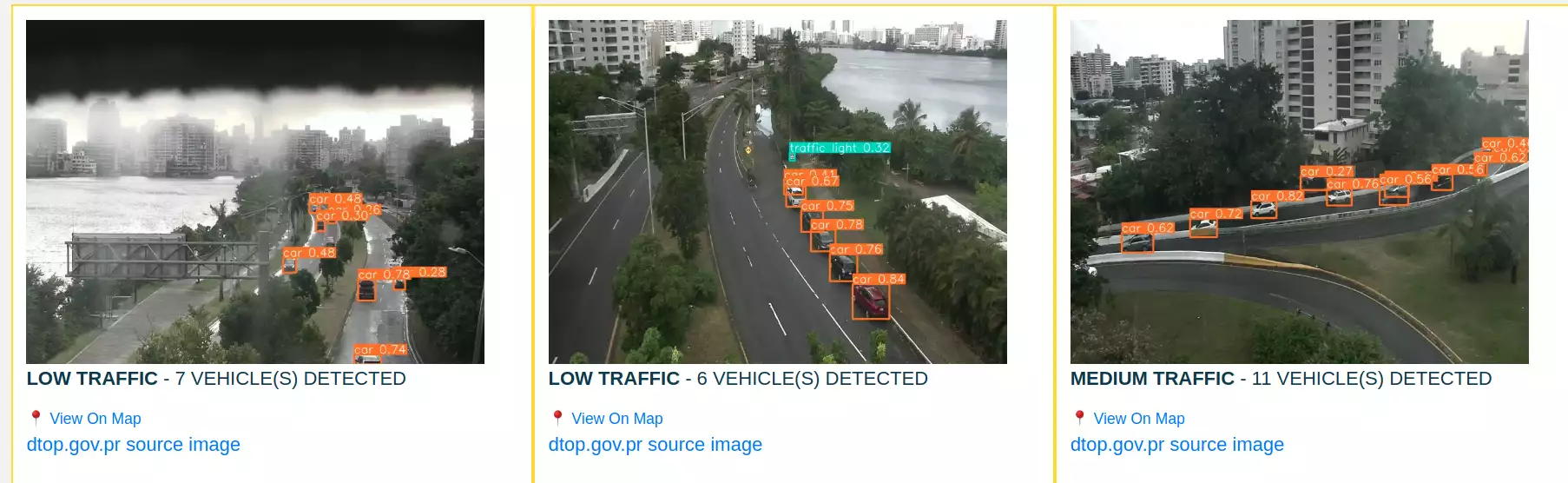### Round 2: The Holistic Approach

Your human brain when viewing the webcam frames doesn't spend it's time counting vehicles and determining a specific number you'd deem as "high" traffic or "low" traffic, so while it works to an impressive degree as a computer it still falls a bit short of great. So how do we do this? Well, perhaps when we were younger it was something closer to counting cars or hearing screams of pain being stuck in heavy traffic that allowed you to correlate the scenerary and level of cars around you with being low/medium/high traffic conditions without you really knowing it, but as we get older we are able to glance at these images of traffic and instantly give someone a reasonable response to what the flow of traffic looks like. We'd like our model to have a similar level of understanding based on entire images versus spending it's time counting the number of cars that are on the road and having to have a human give a fairly arbitrary number to make it's determinations.

Where are we? We now have a semi-accurate way of categorizing images from the webcam and a good idea of the circumstances we are failing to properly detect traffic, and so away we go to write a python script to begin storing all the images that we've retrieved into nicely categorized subfolders based on our yolov5 detection results as they'd be displayed in our application currently. We're going to go back through these afterwards and manually move around the ones we got wrong into their correct subfolders. With a few tweaks to the original code we were using, we're able to get this going quickly.

```                    ```
def getFolder(carCount, route):
if carCount == 0:
return "no"
if carCount < route:
return "low"
if carCount < route:
return "medium"
return "
# save the original and the detection rendered image out to the folder based on our vehicle count.
starttime = time.time()
idx = 1
while True:
for route in sjOriRoutes:
try:
results = model(route)
counts = results.pandas().xyxy.name.value_counts()
vehicleCount = 0
if "car" in counts:
vehicleCount += counts["car"]
if "bus" in counts:
vehicleCount += counts["bus"]
if "truck" in counts:
vehicleCount += counts["truck"]
res = getFolder(vehicleCount, route)
fileName = "results/"+res+"/" + \
time.strftime("%Y%m%d%H%M")+route.split('/')[-1]
im = Image.fromarray(results.imgs)
im.save(fileName)
results.render()
fileName = "detection_results/"+res+"/" + \
time.strftime("%Y%m%d%H%M")+route.split('/')[-1]
im = Image.fromarray(results.imgs)
print(fileName)
im.save(fileName)
except:
continue
print("epoch" + str(idx))
idx += 1
time.sleep(60)
```
```

Running that for a period of time to collect lots of samples from our webcam at different times throughout the day was a great success. A few thousand images produced for each of our categories, despite some of them being wildly wrong we're still optimistic. Opening my results folder in one monitor, a specific subfolder in another with an image viewer I prepared myself for the next tedious hour-or-so of my life.

A few hours of moving images from their original folders into their respective sub-folder by category based on my human understanding of some nuances, I felt confident that most of my training images were correctly labeled. (Perfect is the enemy of progress) So I now wanted to go about building a simple neural network with pytorch that is trained exclusively on my categorized images of the webcams here in puerto rico and see if I was able to meet or exceed the first attempt with using raw object detection counts via yolov5. Easy enough.

```                    ```
from torchvision import transforms
import torch
import urllib
from torchvision.datasets import ImageFolder
from PIL import Image
import numpy as np
import pandas as pd
import seaborn as sns
from tqdm.notebook import tqdm
import matplotlib.pyplot as plt
import torch
import torchvision
import torch.nn as nn
import torch.optim as optim
import torch.nn.functional as F
from torchvision import transforms, utils, datasets
from torch.utils.data import Dataset, DataLoader, SubsetRandomSampler
from sklearn.metrics import classification_report, confusion_matrix
device = torch.device("cuda" if torch.cuda.is_available() else "c
image_transforms = {
"train": transforms.Compose([
transforms.Resize((150,150)),
transforms.RandomHorizontalFlip(),
transforms.ToTensor(),  #0-255 to 0-1, numpy to tensors
transforms.Normalize([0.5,0.5,0.5], # 0-1 to [-1,1] , formula (x-mean)/std
[0.5,0.5,0.5])
]),
"test": transforms.Compose([
transforms.Resize((150,150)),
transforms.RandomHorizontalFlip(),
transforms.ToTensor(),  #0-255 to 0-1, numpy to tensors
transforms.Normalize([0.5,0.5,0.5], # 0-1 to [-1,1] , formula (x-mean)/std
[0.5,0.5,0.5])
])

trainDataset = ImageFolder(root="results-2021-11-16",
transform=image_transforms["trai
valDataset = ImageFolder(root="results-2021-11-16",
transform=image_transforms["test"])
train_count = len(trainDataset)
test_count = len(valDataset)
idx2class = {v: k for k, v in trainDataset.class_to_idx.item
class ConvNet(nn.Module):
def __init__(self,num_classes=126):
super(ConvNet,self).__init__()
#Output size after convolution filter
#((w-f+2P)/s) +1
#Input shape= (256,3,150,150)
#Shape= (256,12,150,150)
self.bn1=nn.BatchNorm2d(num_features=36)
#Shape= (256,12,150,150)
self.relu1=nn.ReLU()
#Shape= (256,12,150,150)
self.pool=nn.MaxPool2d(kernel_size=2)
self.pool=nn.MaxPool2d(kernel_size=2)
#Reduce the image size be factor 2
#Shape= (256,12,75,75)
#Shape= (256,20,75,75)
self.relu2=nn.ReLU()
#Shape= (256,20,75,75)
#Shape= (256,32,75,75)
self.bn3=nn.BatchNorm2d(num_features=32)
#Shape= (256,32,75,75)
self.relu3=nn.ReLU()
#Shape= (256,32,75,75)
self.fc=nn.Linear(in_features=75 * 75 * 32,out_features=num_classes)

def forward(self,input):
output=self.conv1(input)
output=self.bn1(output)
output=self.relu1(output)
output=self.pool(output)
output=self.conv2(output)
output=self.relu2(output)
output=self.conv3(output)
output=self.bn3(output)
output=self.relu3(output)
#Above output will be in matrix form, with shape (256,32,75,75)
output=output.view(-1,32*75*75)
output=self.fc(output)
return ou
model = ConvNet()
model.to(device)
print(model)
loss_function=nn.CrossEntropyLoss()
def multi_acc(y_pred, y_test):
y_pred_softmax = torch.log_softmax(y_pred, dim = 1)
_, y_pred_tags = torch.max(y_pred_softmax, dim = 1)
correct_pred = (y_pred_tags == y_test).float()
acc = correct_pred.sum() / len(correct_pred)
acc = torch.round(acc * 100)
return
# Train it
best_accuracy=0.0
for epoch in range(30):

#Evaluation and training on training dataset
model.train()
train_accuracy=0.0
train_loss=0.0

if torch.cuda.is_available():
images=Variable(images.cuda())
labels=Variable(labels.cuda())

outputs=model(images)
loss=loss_function(outputs,labels)
loss.backward()
optimizer.step()

train_loss+= loss.item()*images.size(0)
_,prediction=torch.max(outputs.data,1)

train_accuracy+=int(torch.sum(prediction==labels.data))

train_accuracy=train_accuracy/train_count
train_loss=train_loss/train_count

# Evaluation on testing dataset
model.eval()

test_accuracy=0.0
if torch.cuda.is_available():
images=Variable(images.cuda())
labels=Variable(labels.cuda())

outputs=model(images)
_,prediction=torch.max(outputs.data,1)
test_accuracy+=int(torch.sum(prediction==labels.data))

test_accuracy=test_accuracy/test_count

print('Epoch: '+str(epoch)+' Train Loss: '+str(train_loss)+' Train Accuracy: '+str(train_accuracy)+' Test Accuracy: '+str(test_accuracy))

#Save the best model
if test_accuracy>best_accuracy:
torch.save(model.state_dict(),'best.model')
best_accuracy=test_accuracy
print('Reached Accuracy: '+ str(best_accuracy))
```
```

Training the model for a long while on my GPU resulted in a 'best.model' file saved for us to use in our application. Let's go ahead and update our original application code that was reliant on our yolov5 model counting vehicles to come up with useful data.

``````cp = torch.load("best_checkpoint.model")
evalModel = ConvNet()
evalModel.eval()
evalModel.to("cud
starttime = time.time()
idx = 1
while True:
finalResult = []
for route in sjOriRoutes:
try:
response = requests.get(route)
image = Image.open(BytesIO(response.content))
image_tensor = inputTransform(image).float()
image_tensor = image_tensor.unsqueeze_(0)
image_tensor = image_tensor.cud
output = evalModel(image_tensor)
_, predicted = torch.max(output, dim=1)
print(idx2class[predicted.item()])
res = idx2class[predicted.item()]
fileName = "static/img/"+route.split('/')[-1]
finalResult.append(
(fileName, route, route, route, route, 0, res, route, route))
image.save(fileName)
except:
conti
with open('latest.json', 'w') as outfile:
json.dump(finalResult, outfile, cls=NpEncoder)
time.sleep(60)
print("epoch" + str(idx))
idx += 1
time.sleep(60)
```
```

And now as you can find at San Juan Puerto Rico we're serving our webcam stills with an educated guess on the flow of traffic on the highways using the DTOP webcams here in Puerto Rico.

Once we confirm our results are on par or better than the original object detection approach, we will substitute yolov5 with our new model in our python script that collects and organizes the training data. The second most exciting part is watching the training data become less and less messy and subsequently getting us better models with less work.

So what's next? We're hoping to get a minimalist training pipeline to have our model become a nightly build based on the training data, if we find our model being more performant than previously, we'll save it out to our file system for our python script to grab and start doing a better job categorizing training data, as well as update our applications models to start using the latest and smartest model available.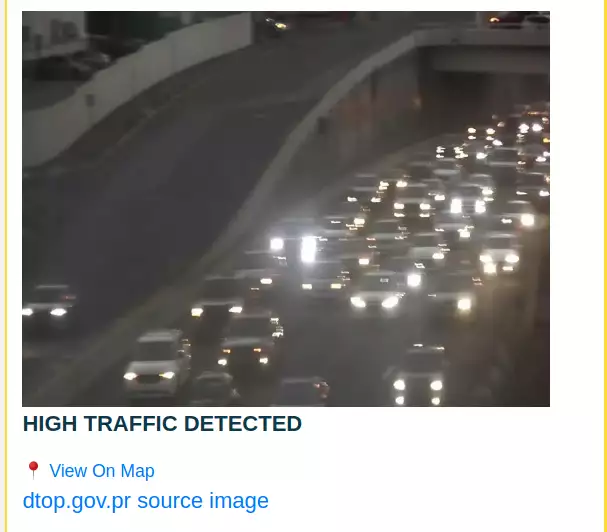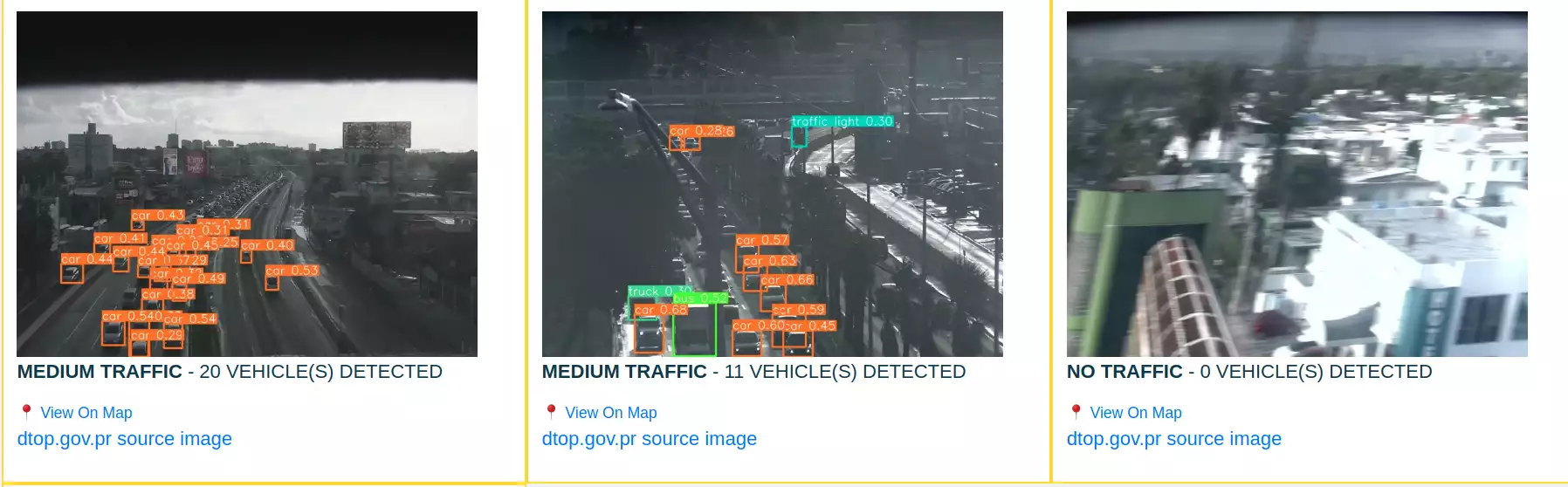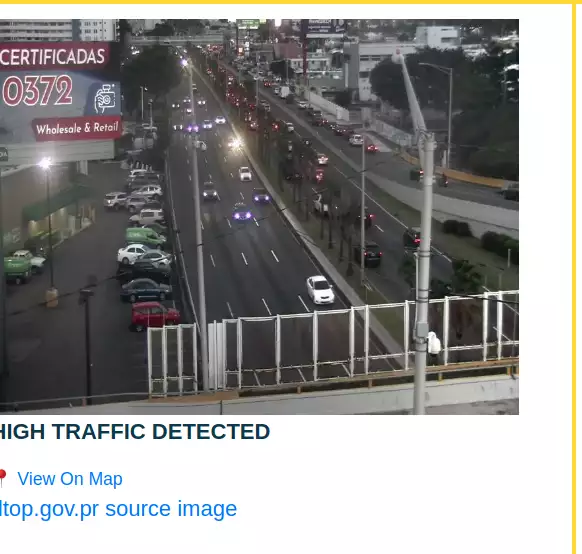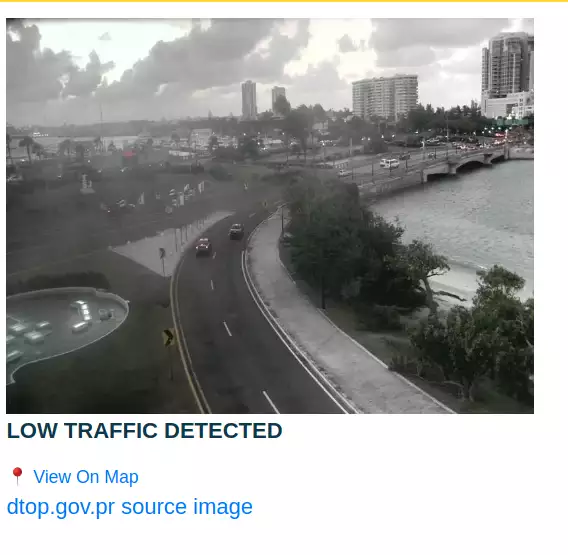Happy Hacking!